# How does Recursion works in C and C++?

## How does Recursion works in C and C++?

In this article, I am going to discuss How does Recursion works in C and C++ in detail. This is a very important topic because a lot of functions or procedures can be written using recursion. That is, they can be written as recursive functions. As part of this article, we are going to discuss the following pointers in detail.

1. What is Recursion?
2. Example of recursion
3. How Recursion Works?
4. Tracing recursion
5. Stack used in Recursion
6. Time complexity
7. Recurrence Relation
##### What is Recursion?

Before understanding Recursion, let us have a look at the below image. Here we have the main function and one more function called function ‘fun’ and that fun function is called by the main function.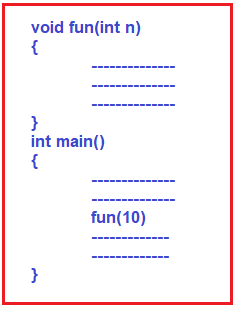First, we need to understand how does this function call is made and how it works. Here, once I start executing the program, it will start executing the program from the main function. First, it will execute the first statement, then it will execute the second statement and then it will execute the third statement i.e. it will call the fun function. Here, the control will move to the fun function definition and start executing that fun function. Inside the fun function, it will start executing the first statement, then the second, and then the third statement. Once it has finished (once the third statement is executed inside the fun function) and the control again comes back to the same line i.e. third line of the main function. If any other operations are present in that line they will execute. Otherwise, it will execute the fourth statement and then the fifth statement.

##### What does it mean by any other operations?

Let say the fun function is returning something and in the main function, I have written added by 2. So, the value of returning from the fun function has to be added by two. So, this addition needs to be done once the function has returned to the main function with some value. Assume that the fun function has a return value of 10. So, 10+2 can be done, only if the fun(10) has returned the value. This is the important point that you should remember for understanding the recursion. For better understanding, please have a look at the below image.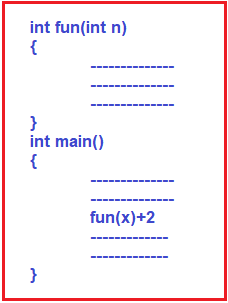With this keep in mind, let us proceed and understand what is a recursive function.

##### What does it mean by Recursive Function?

The general form of recursion is given below.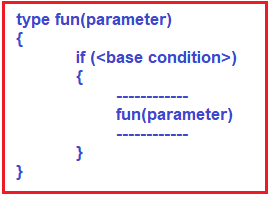This is a recursive function i.e. a function is calling itself. If a function is calling itself then it is called a recursive function. Inside the function body, if you see it is calling itself again, then it is a recursive function.

One important point that you need to remember is, inside recursion, you can see that there is a base condition. So, there must be some base condition that will terminate the recursion. There must be some method to terminate the recursion otherwise it will go into infinite calling. First, we need to call the function the first time, then it will call itself repeatedly again and again. So, there must be some condition at which it must stop.

In this example, the function will call itself as long as the base condition is true or it can stop if the base condition is true. Here if the condition becomes false it will not call further and it stops. So, let us take some examples of the recursive function and study how it works.

##### How does Recursion Work in C and C++?

Let us look at an example to understand how recursion works.

Please have a look at the following code. Here, I have a main function which is having some value in the variable x, and calling function fun1 bypassing that variable ‘X value. The function fun1 that taking parameter ‘n’ which will accept the x value and if the condition is ‘true, it is printing and then calling itself. I have used a C language code here. So, it’s printing and again calling itself for a reduced value of n.

```void fun1(int n)
{
if(n>0)
{
printf("%d ",n);
fun1(n-1);
}
}
void main()
{
int x=3;
fun1(x);
}
```

Output: 3 2 1

In the above example, we are passing 3 to the fun1 function from the main function. Let us see what will be the result and how it works? Let’s, trace this recursive function and check.

##### How to trace a Recursive Function?

A recursive function is traced in the form of a tree. So, let us start tracing the above example. When the condition is true inside the fun1 function there are two statements to be executed. In the first statement, it will print the n value and in the second statement it will call itself bypassing (n-1) and this has to be done only when n is greater than 0.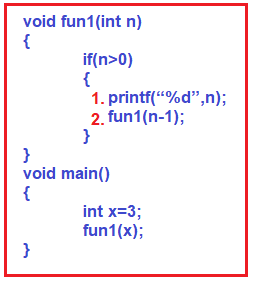Let’s start tracing, from the main function, we are calling function fun1 bypassing ‘X i.e. value 3. So, the first time it has got 3, and 3 is greater than 0 and hence the condition becomes true. So, the first step is to print ‘n’ and the second step is to call itself again fun1 for 3-1 i.e. 2. Here, the fun1(3) call has not been completed. It calling itself again.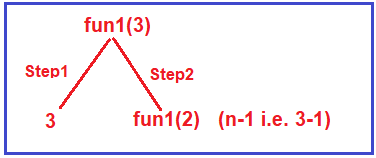So, it will call itself again bypassing fun1(2). So, let us execute fun1(2), again it will start, 2 is greater than ‘0’ and hence the condition becomes true. So, the first step is to print 2 and then call itself again bypassing fun1(1). Now, the fun1(2) call has not finished, it has printed 2 and it has to call fun1(1).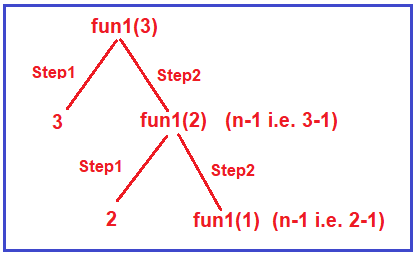So again, a new call, a fresh call, that fresh call is fun1(1). 1 is greater than 0, so we have to perform the two steps. The first step is to print 1 and then call itself by passing fun1(0). Now, the fun1(1) call has not finished, it has printed 1 and it has to call fun1(0).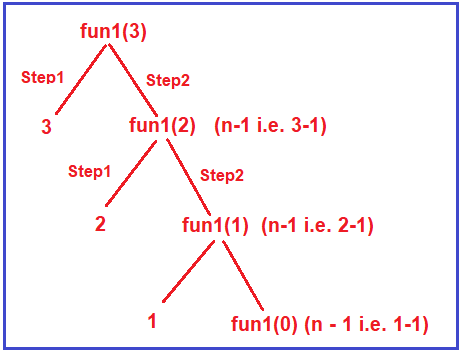Now, fun1(0), 0 is greater than 0, no it’s not greater than 0. So, it will not enter inside, it will not perform those two steps and it does nothing. So, no printing and no calling and it will not enter inside and it will come outside of the function. So, the fun1(0) call doesn’t do anything. So, it will go back to the previous function call and so on and finally come out from the fun1 to the main function where it is initially called. So, a recursive function forms a tree and this is called a tracing tree of a recursive function. Now we will take one more example.

##### Example:

Please have a look at the below example which is also an example of the recursive function.

```void fun2(int n)
{
if(n>0)
{
fun2(n-1);
printf("%d",n);
}
}
void main()
{
int x=3;
fun2(x);
}
```

The above example is very similar to the first example. Let me compare both the example and show you the difference.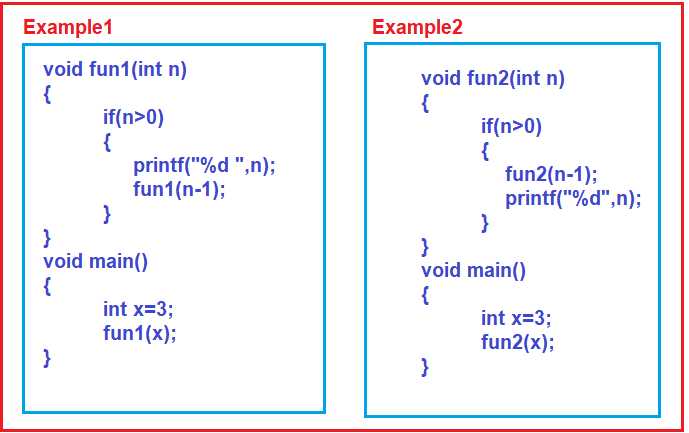If you look at the main function of both the example, they are having one variable called x and calling one function (Example1 calling fun1 function and Example2 calling fun2 function) bypassing that x value.

The difference in both the example is that, in example 1 inside the fun2 function if the condition is true (i.e. n > 0), first it is printing the n value and then calling itself but in example 2, first it is calling itself, and then printing the n value, then what will be the output. Let’s trace example 2 and find out the output.

The program execution will start from the main function. The main function calling the function fun2 by passing value 3 i.e. fun2(3). Inside the fun2 function, first, it will check whether n > 0 and here, n is 3, so 3 is greater than 0 and the condition is satisfied. The first statement within the if block is executed i.e. it calls the fun2 function by passing n-1 i.e. 2. What about the second statement i.e. printing? It will not be executed at this point in time. The point that you need to remember is first the first statement has to be finished in order to execute the second statement i.e. printing. For better understanding please have a look at the below image.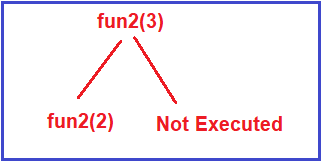Let us take fun2(2) call, with n=2, the condition again satisfied as 2 is greater than 0. Again, two steps, first it will call fun2 with n-1 i.e. it will call itself for fun2(1) and the second statement will not be executed at this point. Once the first statement is finished, the second statement will execute. At this point, the tracing tree will be like below.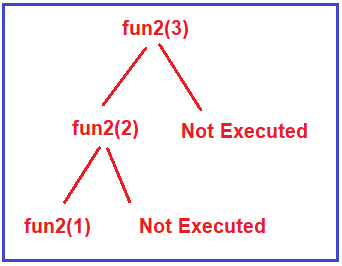Let us trace fun2(1). Again 1 is greater than 0 and hence the condition is satisfied and again two steps. In the first step, it will call itself bypassing n-1 i.e. fun2(0), and similarly, the second statement will only execute once the first statement completes its execution. So, at this point, the tracing tree of this recursive function is like the below.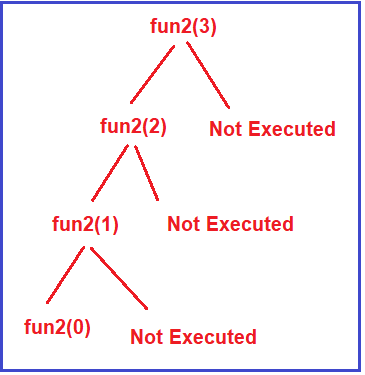The next call is fun2(0). Now fun2(0), 0 is greater than 0, no. So, it will not enter inside this ‘if’ block and it will come out i.e. it does nothing. So, this call with parameter 0 has terminated.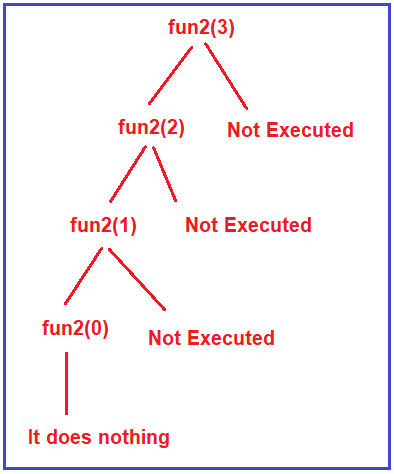Now once this call has been terminated the control should go back to the previous call. The previous call was fun2(1), it will go back to the function call and execute the next statement i.e. the second statement which is nothing but to print the n value. At this call, the n value is 1 and hence it will print 1. For better understanding, please have a look at the below image.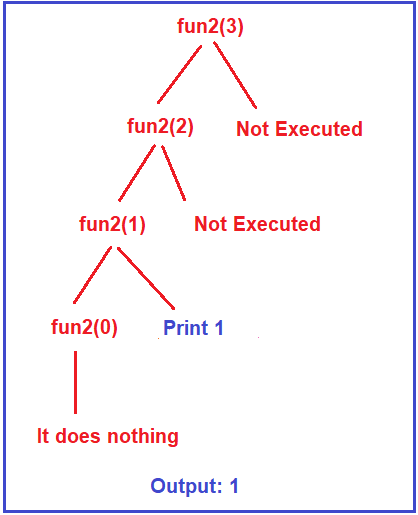Then it will go back to the previous call i.e. fun2(2), and the second thing that is remaining here is printing, so the value 2 is printed then it will come out of this function and finish. For better understanding, please have a look at the following image.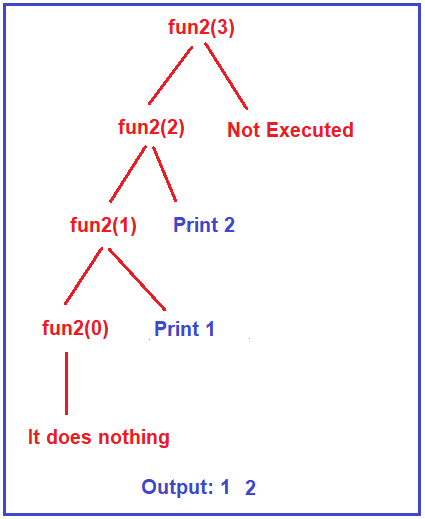Once the fun2(2) call finishes, it goes back to the previous call i.e. fun2(3), and the second thing that is remaining here is printing, so the value 3 is printed. And the output you will get from this function is 1 2 3 as shown in the below image.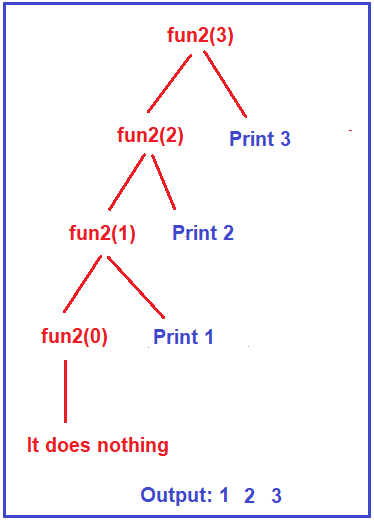The output of example 1 was 3, 2, 1 and the output of example 2 is 1, 2, 3.

Now, let us compare both of the examples, in example 1, first, the printing was done and then the recursive call was made but in example 2, first the recursive call was made and then the printing was done at returning time.

Note: The most important thing that you need to understand in recursion is that recursion has two phases. One is the calling phase and the other one is returning phase.

##### Real-Time Example of Recursion:

Now, let us take one example and see the differences between them. Suppose there is a room and in that room, there is a bulb and a door. From that room, you can enter into one more room, and again in that room, there is a door and there is also a bulb fixed in it. Again, from that room, you can enter into one more room and in that room also there is a door and a fixed bulb. So, there are a total of 3 rooms i.e. room 1, room 2, room 3 and each room is having a bulb and a door.

Now if I give you the following two instructions,

1. Switch on the bulb,
2. Go to the next room.

Now if I make you stand in room 1 and ask you to perform the above two 2 steps recursively, then what will you do. Let us check. In the first room, you will switch on the bulb i.e. first step, the second step you will go to the next room i.e. 2. So, the first bulb that you have switched on.

Then you will enter into next room i.e. room 2 and again what you will do is recursively is switch on the bulb i.e. second bulb and go to the third room. Similarly, in the third room, recursively you will switch on the bulb i.e. bulb 3, and go to the next room. Now, there is no next room further. So, it was more like a recursive call, a function calling itself again and again. This is termination there is no next room. What do you do now?

You will definitely come out of those rooms. Your work has finished. So, from the third room, you will come back to the second room. Now, will you do anything in the 2nd room? No, you’ll simply come out from the 2nd room to the 1st room and you are also not going to do anything in the 1st room. So, you will come out from the first room also.

So, third room to second room, second room to first room and then you’ll simply come out. It means the first step was you were entering into the rooms; it was just like calling. Once you have into the third room, you cannot go further. So, you have to return back, this is nothing but returning.

So, you have gone through two phases. One is the calling phase and another one is returning phase or we can say that entering one is ascending and returning one is descending. So, you are going to next, next, next ascending and then you came back, back, back, so descending. So, you have done ascending and descending and, in this example, descending time we have not done anything.

##### Another Example of Recursion:

Now we will discuss one more example. Now, for the same three rooms, I have given the following instructions to perform

1. Go to the next room.
2. Switch on bulb

The first step is going to the next room and the second step is to switch on the bulb. These are the two steps. We have changed the order of the previous example. Now let us see if these steps are performed then what will be the result.

First, you are in the first room and I have given you two instructions, go to the next room and switch on the bulb. So, what you will do is you will not switch on the bulb, you will simply go to the next room i.e. room 2 and again in room 2, you will not switch on the bulb but you will move to the next room i.e. room 3 and in room 3 first you will move to the next room but there is no next room and hence from here only you will perform the second step i.e. switch on the bulb on room 3. So, the first bulb to be switched on is the third bulb. Then you will come back to the previous room i.e. room 2 and switch on the second bulb and again you’ll come back to the first room and switch on the first bulb.

So, I have two different versions of the steps here, In the first example the steps are as follows:

1. Switch on the bulb.
2. Go to the next room.

In this example, Step 2 is the recursive call, first perform the operation then the recursive call. In the second example, the following are the steps:

1. Go to the next room.
2. Switch on the bulb.

In this example, Step 1 is the recursive call, first perform the recursive call then the operation.

##### Generalized Form of Recursive Function:

The most important thing that you have to remember is, recursion has two phases. One is the calling phase and the other one is returning phase. Let us understand the generalized form of a recursive function. Please have a look at the below image.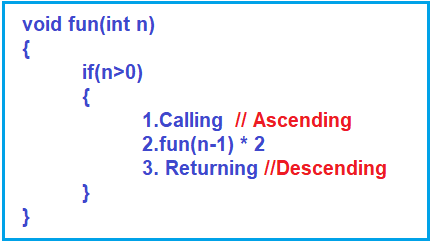In the recursive function call, before the function call if anything is there then that will be executed at calling time and after the function call if anything is there that will be executed at returning time. We can call statement 1 ascending and statement 3 descending. So, the recursive function has two phases that are ascending and descending.

##### Difference between Recursion and Loop?

Let us compare recursion with loop. Loops are repeating statements then what is recursion? Recursion is also repeating i.e. calling the same function again and again. So, is not it repeating? Yes, recursion is also just like repeating. But the difference is a loop will have only ascending phase but the recursion will have both ascending as well as descending phases. That is the strength and power of recursion that you can utilize or exploit for writing code easier.

In the next article, I am going to discuss How Recursion uses Stack with Example. Here, in this article, I try to explain How does Recursive Function Work in C and C++ with Examples and I hope you enjoy this How Recursion Works article. Please give your feedback and suggestions about this How Recursion Works article.